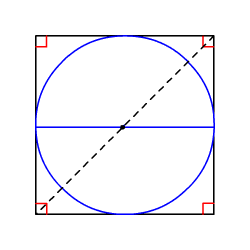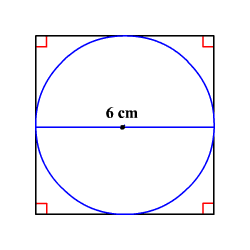# Circles Inscribed in Squares

When a circle is inscribed in a square , the diameter of the circle is equal to the side length of the square.You can find the perimeter and area of the square, when at least one measure of the circle or the square is given.

For a square with side length $s$ , the following formulas are used.

Perimeter = $4s$

Area = ${s}^{2}$

Diagonal = $s\sqrt{2}$

Similarly, you can find the circumference and area of the circle , when at least one measure of the circle or the square is given.

For a circle with radius $r$ , the following formulas are used.

Circumference = $2\pi r$

Area = $\pi {r}^{2}$

Example 1:

Find the perimeter of the square.When a circle is inscribed in a square, the diameter of the circle is equal to the side length of the square.

So, the side length of the square is $6$ cm.

The perimeter $P$ of a square with side length $s$ is given by $P=4s$ .

Substitute $6$ for $s$ in $P=4s$ .

$\begin{array}{l}P=4\left(6\right)\\ \text{\hspace{0.17em}}\text{\hspace{0.17em}}\text{\hspace{0.17em}}\text{\hspace{0.17em}}\text{\hspace{0.17em}}=24\end{array}$

The perimeter of the square is $24$ cm.

Example 2:

What is the area of a circle that is inscribed in a square of area $64$ square units?

When a circle is inscribed in a square, the length of each side of the square is equal to the diameter of the circle. That is, the diameter of the inscribed circle is $8$ units and therefore the radius is $4$ units.

The area of a circle of radius $r$ units is $A=\pi {r}^{2}$ .

Substitute $r=4$ in the formula.

$\begin{array}{l}A=\pi {\left(4\right)}^{2}\\ \text{\hspace{0.17em}}\text{\hspace{0.17em}}\text{\hspace{0.17em}}\text{\hspace{0.17em}}\text{\hspace{0.17em}}=16\pi \\ \text{\hspace{0.17em}}\text{\hspace{0.17em}}\text{\hspace{0.17em}}\text{\hspace{0.17em}}\text{\hspace{0.17em}}\approx 50.24\end{array}$

Therefore, the area of the inscribe circle is about $50.24$ square units.